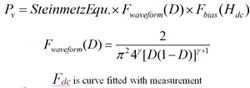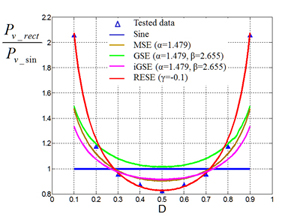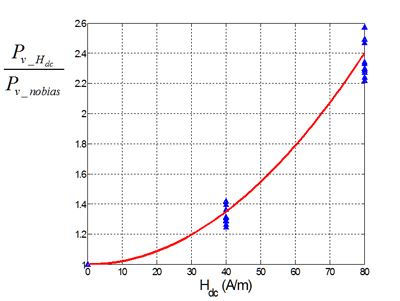Contact Us
LIBRARY

# Core loss Model for Rectangular AC Voltages and DC Bias CurrentFor most power converters, the voltage waveforms are mostly rectangular or composed of rectangular shapes. The waveform will have an impact on the value of the core loss. And for power inductor, the core will usually be biased by a DC current. The DC bias will affect the core loss. Generally, the core loss will increase by several fold if the bias is strong. An accurate model is necessary in order to predict the core loss under such excitations and assist in the design trade-offs. The most popular core loss models now are the modified Steinmetz equation (MSE), the generalized Steinmetz equation (GSE) and the improved generalized Steinmetz equation (iGSE). However, the measurement data shows these models don't give accurate prediction. A new model is proposed to improve the accuracy for the core loss under rectangular AC voltages and DC bias current by employing a parameter and DC bias factor, which depends on the material, frequency and temperature. Several ferrite materials are characterized and modeled.Fig. 1. (a) Test data and various models of 3F35 MnZn ferrite: Waveform impact (500kHz, RT).Fig. 1. (b) DC bias factor (500kHz, RT).
INDUSTRY PARTNERS